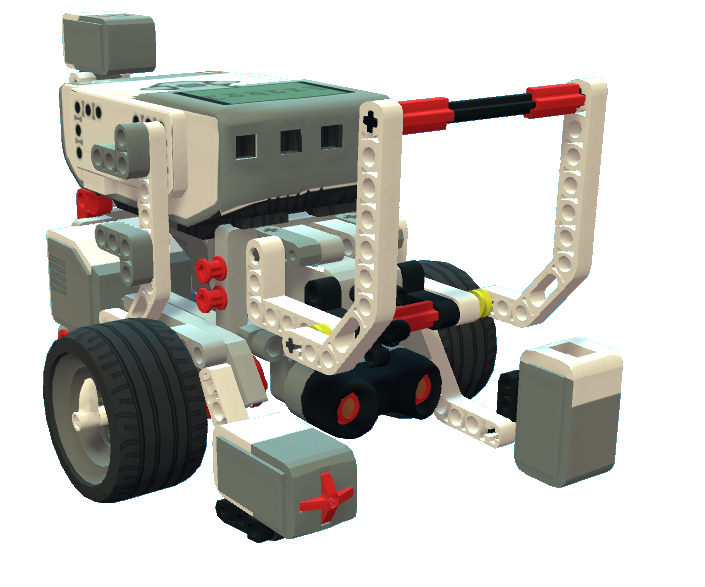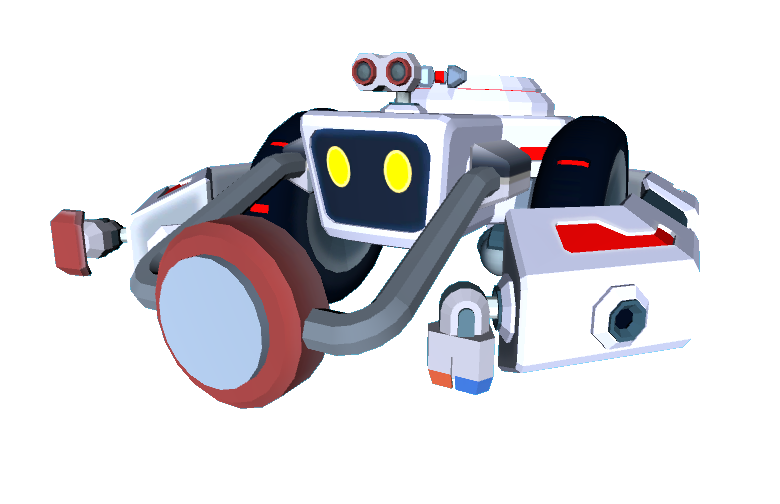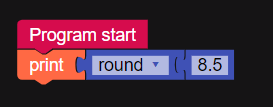Round BlockThis block works for both EV3 Robot and Ruby Robot!This block will round a number with a decimal, to a whole number. If the number after the decimal is lower than 5, the number will be rounded down. If the number after the decimal is 5 or higher, the number will be rounded up to the next whole number.

For example 8.3 will become 8.

8.5, 8.8 and 8.9 will become 9.

Example Program

This program prints 9 to the console: